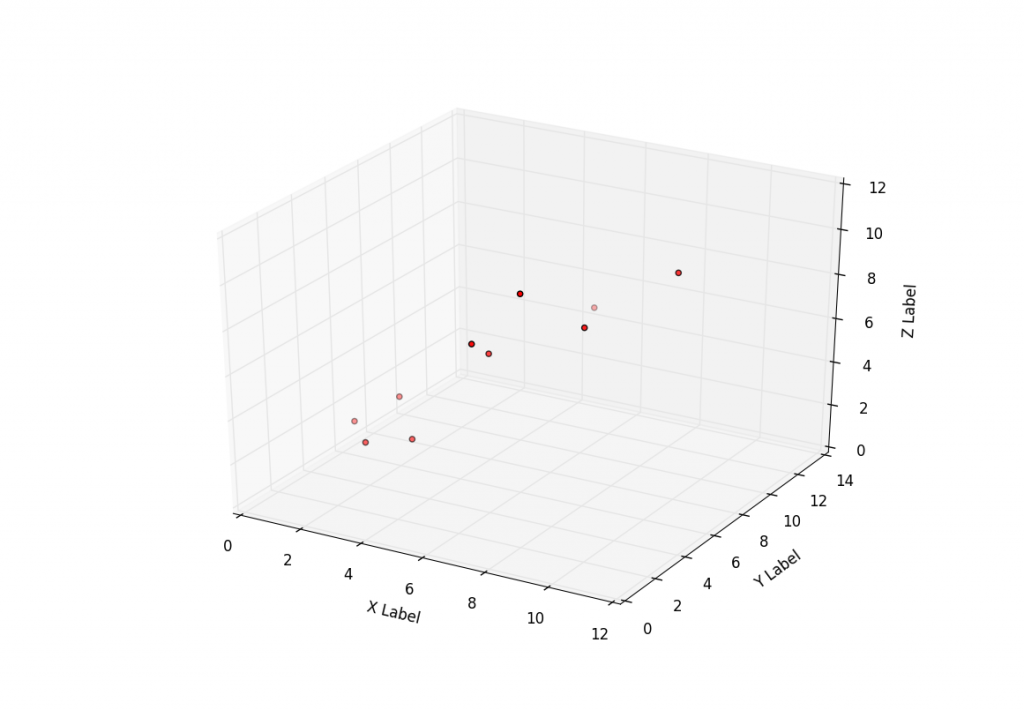## 3D Scatter Plot with Python and Matplotlib

Besides 3D wires, and planes, one of the most popular 3-dimensional graph types is 3D scatter plots. The idea of 3D scatter plots is that you can compare 3 characteristics of a data set instead of two.

This tutorial covers how to do just that with some simple sample data. Here is the code that generates a basic 3D scatter plot that goes with the video tutorial:

```from mpl_toolkits.mplot3d import Axes3D
import matplotlib.pyplot as plt

fig = plt.figure()

x =[1,2,3,4,5,6,7,8,9,10]
y =[5,6,2,3,13,4,1,2,4,8]
z =[2,3,3,3,5,7,9,11,9,10]

ax.scatter(x, y, z, c='r', marker='o')

ax.set_xlabel('X Label')
ax.set_ylabel('Y Label')
ax.set_zlabel('Z Label')

plt.show()
```

The next tutorial:• Matplotlib Crash Course

• 3D graphs in Matplotlib

• 3D Scatter Plot with Python and Matplotlib
• More 3D scatter-plotting with custom colors

• 3D Barcharts

• 3D Plane wireframe Graph

• Live Updating Graphs with Matplotlib Tutorial

• Modify Data Granularity for Graphing Data

• Geographical Plotting with Basemap and Python p. 1

• Geographical Plotting with Basemap and Python p. 2

• Geographical Plotting with Basemap and Python p. 3

• Geographical Plotting with Basemap and Python p. 4

• Geographical Plotting with Basemap and Python p. 5

• Advanced Matplotlib Series (videos and ending source only)